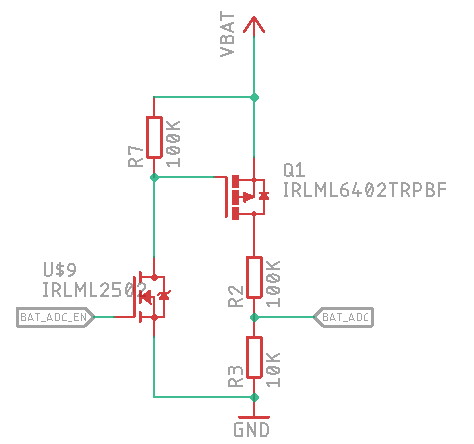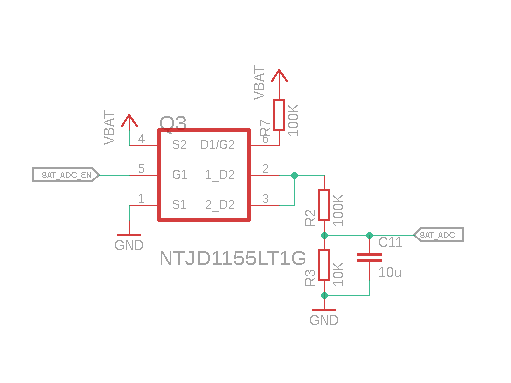Andrey Ovcharov

Professional Software Engineer and hobbyist Hardware enthusiast

# How to measure battery level with ESP32 microcontroller

Autonomous devices like URU Key require periodic measurement of the battery voltage. I am going to use very simple schematics and very simple algorithm to understand how much juice my battery has at the moment.

There is a great thread about measurement circuitry and a switchable voltage divider on StackOverflow. Following the thread I have created the following circuitry for the battery measurement:The schematics uses two MOSFETs to enable measurement circuitry on demand and prevent leaking the power trough the resistors.

Another option I have found was discussed at this thread. Guys offered to use NTJD1155L combined MOSFETs to save space on the PCB. The corresponding schematics looks as follows:The positive battery terminal is connected to the line `VBAT`, the ADC of ESP32 is connected to the `BAT_ADC` line and the line `BAT_ADC_EN` is used to enable and disable the measurement circuitry.

Let’s write the code which reads the value from ADC and converts it to the battery level in the range from 0 to 100%.

In my device I have used resistors of 100K and 10K, the ones I have at the moment:

``````#define R2 100
#define R3 10
``````

The output voltage of the resistor divider is calculated as `Vout = (Vin * R3) / (R2 + R3)`.

``````#define VOLTAGE_OUT(Vin) (((Vin) * R3) / (R2 + R3))
``````

As a very rough estimation, we can take the battery voltage of 4.2V as 100% and the voltage of 3.3V as 0%. I will convert them to millivolts to avoid floating-point calculations.

``````#define VOLTAGE_MAX 4200
#define VOLTAGE_MIN 3300
``````

The reference voltage of ESP32 ADC is 1100mV. Let’s define it:

``````#define ADC_REFERENCE 1100
``````

The value returned from ADC working in 12-bit mode will be in range 0 to 4095. Then we can convert the voltage to ADC value using the following formula:

``````#define VOLTAGE_TO_ADC(in) ((ADC_REFERENCE * (in)) / 4096)
``````

So, the minimal and maximal values of battery voltage converted by a resistor divider and returned from ADC are:

``````#define BATTERY_MAX_ADC VOLTAGE_TO_ADC(VOLTAGE_OUT(VOLTAGE_MAX))
``````

Retrieving value from ADC is pretty straightforward and well described in official Espressif documentation. Let’s say we have the value from ADC in the variable called `adc`. Then the battery level calculation will look as follows:

``````int calc_battery_percentage(int adc)
{
To start measurement we have to enable a high level on the GPIO port connected to the `BAT_ADC_EN` line. Then the current battery level is read from ADC and converted to the percentage value. After the measurement is done, the GPIO port should be set to a low level to avoid battery drain through the divider.